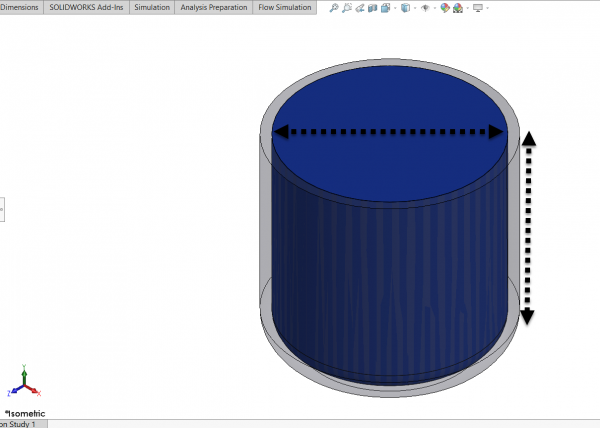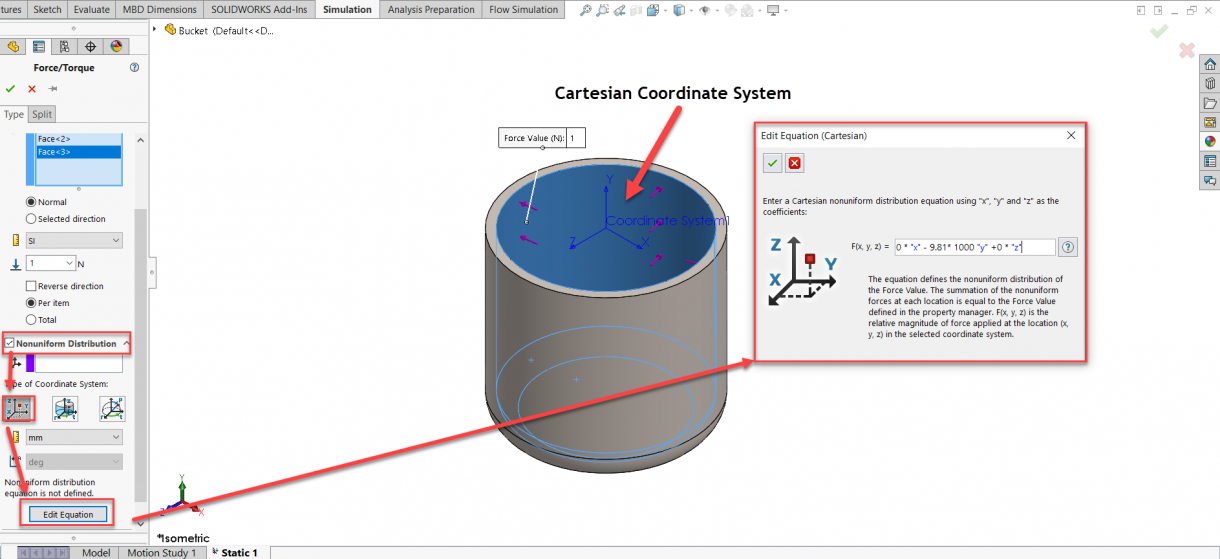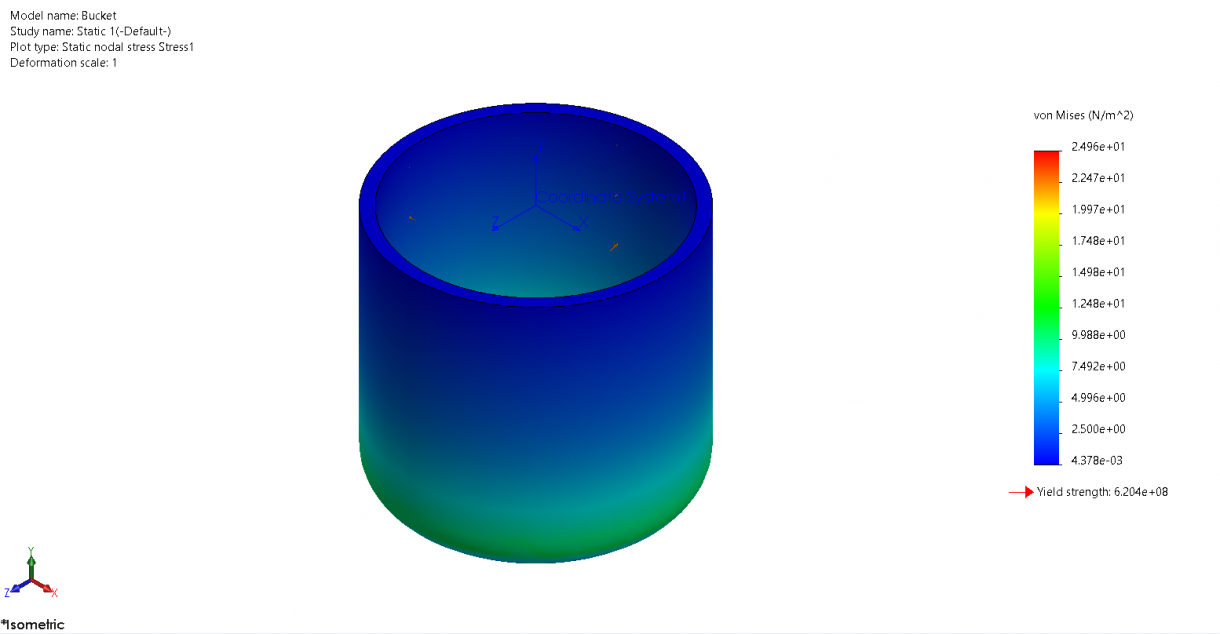# Simulating Hydrostatic Pressure in SOLIDWORKS SimulationArticle by Mersedeh Zandvakili updated June 22, 2020

###### Article

SOLIDWORKS Simulation lets you create studies that review the effects of hydrostatic pressure of a fluid in a model. The displacements and stresses caused by hydrostatic pressures can be calculated by applying non-uniform force distribution. In the example below, the stresses caused by hydrostatic pressure in a bucket filled with water are investigated. The height and the diameter of the bucket is one meter.Bucket Filled Completely with Water

A static simulation study is created and the bucket is fully constrained at the bottom of the bucket. Although the model is self-equilibrated as all the forces cancel each other, the fixtures are still required to account for numerical errors.

To apply the hydrostatic pressure to the bucket, a non-uniform force needs to be applied to all the inner faces of the bucket. A non-uniform force distribution is applied to these faces using an equation. To define the equation, a Cartesian coordinate system is used. For simplicity, a coordinate system at the top level of water is being created and used in defining the force equation.Applying a Non-uniform Force Distribution

The coefficients of the X and Z components are set to zero so that there is no force in these directions. However, in the Y direction (which is the direction of hydrostatic pressure), a coefficient of ρ * g (density of water multiplied by the gravitational constant) is multiplied by the height of water. That means that at the surface of water, the hydrostatic pressure is zero and as we move down through the depth of fluid, the pressure increases linearly with the depth.Von Mises Stresses Due to Hydrostatic Pressure of Water in a Bucket

#### Get Certified SOLIDWORKS Services from Javelin

Javelin Experts can help you to:

### Posts related to 'Simulating Hydrostatic Pressure in SOLIDWORKS Simulation'

#### Find Related Content by TAG:### Mersedeh Zandvakili

Mersedeh Zandvakili is a SOLIDWORKS Technical Support Applications Expert, based in the Javelin Oakville Head Office.